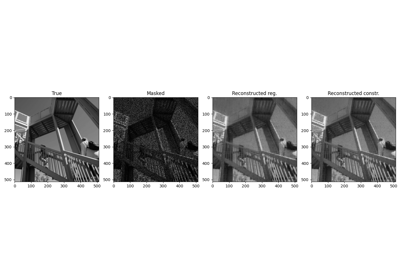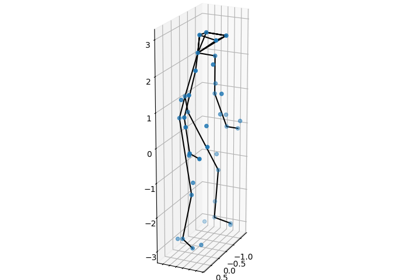# pyproximal.Nuclear#

class pyproximal.Nuclear(dim, sigma=1.0)[source]#

Nuclear norm proximal operator.

The nuclear norm is defined as $$\sigma\|\mathbf{X}\|_* = \sigma \sum_i \lambda_i$$ where $$\mathbf{X}$$ is a matrix of size $$M \times N$$ and $$\lambda_i$$ is the i:th singular value of $$\mathbf{X}$$, where $$i=1,\ldots, \min(M, N)$$.

The weighted nuclear norm, with the positive weight vector $$\boldsymbol\sigma$$, is defined as

$\|\mathbf{X}|\|_{{\boldsymbol\sigma},*} = \sum_i \sigma_i\lambda_i(\mathbf{X}) .$
Parameters
dimtuple

Size of matrix $$\mathbf{X}$$

sigmafloat or numpy.ndarray, optional

Multiplicative coefficient of the nuclear norm penalty. If sigma is a float the same penalty is applied for all singular values. If instead sigma is an array the weight sigma[i] will be applied to the i:th singular value. This is often referred to as the weighted nuclear norm.

Notes

The nuclear norm proximal operator is:

$\prox_{\tau \sigma \|\cdot\|_*}(\mathbf{X}) = \mathbf{U} \diag \{ \prox_{\tau \sigma \|\cdot\|_1}(\boldsymbol\lambda) \} \mathbf{V}^H$

where $$\mathbf{U}$$, $$\boldsymbol\lambda$$, and $$\mathbf{V}$$ define the SVD of $$X$$.

The weighted nuclear norm is convex if the sequence $$\{\sigma_i\}_i$$ is non-ascending, but is in general non-convex; however, when the weights are non-descending it can be shown that applying the soft-thresholding operator on the singular values still yields a fixed point (w. r. t. a specific algorithm), see  for details.

1

Gu et al. “Weighted Nuclear Norm Minimization with Application to Image Denoising”, In the IEEE Conference on Computer Vision and Pattern Recognition, 2862-2869, 2014.

Methods

 __init__(dim[, sigma]) affine_addition(v) Affine addition chain(g) Chain grad(x) Compute gradient postcomposition(sigma) Postcomposition precomposition(a, b) Precomposition prox(**kwargs) proxdual(**kwargs)

## Examples using pyproximal.Nuclear#Low-Rank completion via SVD

Low-Rank completion via SVDNon-rigid structure-from-motion (NRSfM)

Non-rigid structure-from-motion (NRSfM)